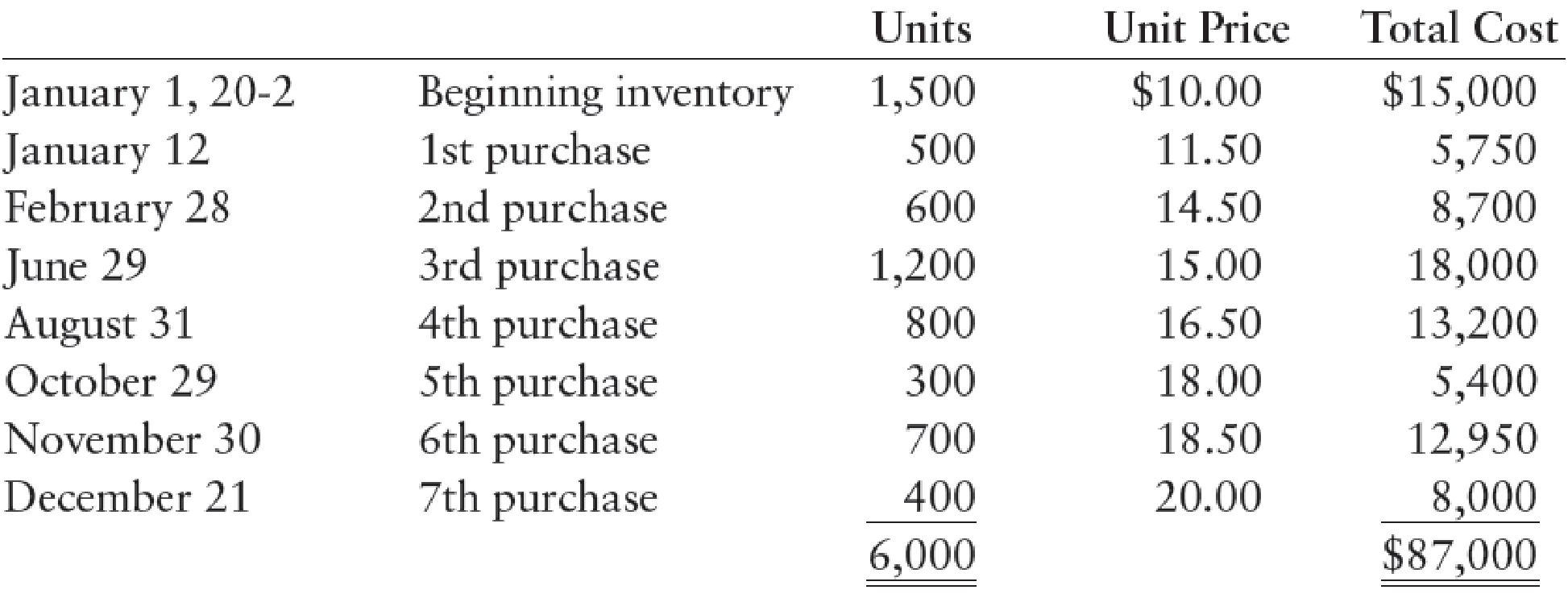Chapter 13, Problem 1MP

Chapter
Section
Textbook Problem

Hurst Company’s beginning inventory and purchases during the fiscal year ended December 31, 20-2, were as follows:There are 1,200 units of inventory on hand on December 31, 20-2.REQUIRED 1. Calculate the total amount to be assigned to the cost of goods sold for 20-2 and ending inventory on December 31 under each of the following periodic inventory methods: (a) FIFO (b) LIFO (c) Weighted-average (round calculations to two decimal places) 2. Assume that the market price per unit (cost to replace) of Hurst’s inventory on December 31 was $18. Calculate the total amount to be assigned to the ending inventory on December 31 under each of the following methods: (a) FIFO lower-of-cost-or-market (b) Weighted-average lower-of-cost-or-market 3. In addition to taking a physical inventory on December 31, Hurst decides to estimate the ending inventory and cost of goods sold. During the fiscal year ended December 31, 20-2, net sales of$100,000 were made at a normal gross profit rate of 35%. Use the gross profit method to estimate the cost of goods sold for the fiscal year ended December 31 and the inventory on December 31.

1.

(a)

To determine

Calculate the total amount of cost of goods sold and cost of ending inventory on December 31, under FIFO method (Periodic inventory system).

Explanation

First-in-First-Out (FIFO): In First-in-First-Out method, the first purchased items are sold first. The value of the ending inventory consists of the recently purchased items.

Calculate the total amount of cost of goods sold and cost of e...

2.

(b)

To determine

Calculate the total amount of cost of goods sold and cost of ending inventory on December 31, under LIFO method (Periodic inventory system).

3.

(c)

To determine

Calculate the total amount of cost of goods sold and cost of ending inventory on December 31, 20-2 under weighted average cost method.

2.

(a)

To determine

Calculate the cost of ending inventory on December 31 under FIFO method (Lower of cost or market).

2.

(b)

To determine

Calculate the cost of ending inventory on December 31 under weighted average cost method (Lower of cost or market).

3.

To determine

Estimate the cost of goods sold and ending inventory for the year December 31 uing the gross profit methods.

Still sussing out bartleby?

Check out a sample textbook solution.

See a sample solution

The Solution to Your Study Problems

Bartleby provides explanations to thousands of textbook problems written by our experts, many with advanced degrees!

Get Started

List the major activities in operations management.

Foundations of Business (MindTap Course List)

Why do economists make assumptions?

Essentials of Economics (MindTap Course List)

What are reversing entries, and why are they used?

Intermediate Accounting: Reporting And Analysis

If you were the CFO of a company that had to decide on hundreds of potential projects every year, would you wan...

Fundamentals of Financial Management, Concise Edition (with Thomson ONE - Business School Edition, 1 term (6 months) Printed Access Card) (MindTap Course List)

Why should policymakers care about GDP?

Principles of Macroeconomics (MindTap Course List)